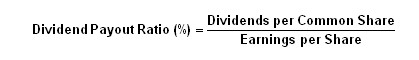# Dividend Payout Ratio

Rahul Gupta , 24 April 2021

This ratio identifies the percentage of earnings (net income) per common share allocated to paying cash dividends to shareholders. The dividend payout ratio is an indicator of how well earnings support the dividend payment.

Here's how dividends "start" and "end." During a fiscal year quarter, a company's board of directors declares a dividend. This event triggers the posting of a current liability for "dividends payable." At the end of the quarter, net income is credited to a company's retained earnings, and assuming there's sufficient cash on hand and/or from current operating cash flow, the dividend is paid out. This reduces cash, and the dividends payable liability is eliminated.

The payment of a cash dividend is recorded in the statement of cash flows under the "financing activities" section.

Formula:Components:
Note:
Zimmer Holdings does not pay a dividend. An assumed dividend amount, as of December 31, 2005, is provided to illustrate the ratio's calculation:

 0.80 ÷ 2.96 = 27%

The numerator represents the annual dividend per share paid in cash and the denominator (income statement) represents the net income per share.

Variations:
At the bottom of the income statement, after the stated amount for net income (net earnings), the per share amounts for "basic" net income per common share and "diluted" net income per common share are provided. The basic per share amount does not take into consideration the possible effects of stock options, which would increase the number of shares outstanding. The diluted per share amount does take into account precisely this possible dilution. Conservative analysis would use the diluted net income per share figure in the denominator.

In another version of the dividend payout ratio, total amounts are used rather than per share amounts. Nevertheless, an investor should arrive at the same ratio percentage.

Note: In the U.K. there is a similar dividend payout ratio, which is known as "dividend cover". It's calculated using earnings per share divided by dividends per share.

Commentary:
Our first observation states the obvious - you only use this ratio with dividend-paying companies. Investors in dividend-paying stocks like to see consistent and/or gradually increasing dividend payout ratios. It should also be noted that exaggerated (i.e. very high) dividend ratios should be looked at skeptically.

The question to ask is: Can the level of dividends be sustained? Many investors are initially attracted to high dividend-paying stocks, only to be disappointed down the road by a substantial dividend reduction (see remarks below). If this circumstance happens, the stock's price most likely will take a hit.

Secondly, dividend payout ratios vary widely among companies. Stable, large, mature companies (i.e. public utilities and "blue chips") tend to have larger dividend payouts. Growth-oriented companies tend to keep their cash for expansion purposes, have modest payout ratios or choose not to pay dividends.

Lastly, investors need to remember that dividends actually get paid with cash - not earnings. From the definition of this ratio, some investors may assume that dividend payouts imply that earnings represent cash, however, with accrual accounting, they do not. A company will not be able to pay a cash dividend, even with an adequate unrestricted balance in retained earnings, unless it has adequate cash.

In view of this accounting treatment of dividends, it is incumbent upon investors to check a company's dividend payout ratio against an adequate margin of free cash flow to ensure that the payout percentage (ratio) is sustainable.

Category Students, Other Articles by - Rahul Gupta